Time: 3 Hours                                                                                                     Max. Marks: 100

NOTE: There are 9 Questions in all.

·      Question 1 is compulsory and carries 20 marks. Answer to Q. 1. must be written in the space provided for it in the answer book supplied and nowhere else.

·      Out of the remaining EIGHT Questions answer any FIVE Questions. Each question carries 16 marks.

·      Any required data not explicitly given, may be suitably assumed and stated.

Q.1       Choose the correct or best alternative in the following:                                         (2x10)

a.       When a low resistance is connected in parallel with a high resistance, the combined resistance is

(A)  Always more than the high resistance.

(B)  Always less than the low resistance.

(C)    Always between the high resistance & low resistance.

(D)   Either lower or higher than low resistance depending on the value of high resistance.

b.      Q factor of an inductive coil is given by

(A) R/Z                                               (B)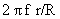(C)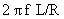(D) l r/l

c.   The r.m.s. value of sinusoidal 100 V peak to peak is _________ volt.

(A)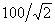(B)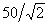(C)  50                                                (D)  100

d.   If the readings of the two wattmeters in the 2-wattmeter method of power measurement are 4.5 kW and 3.5 kW respectively and the latter reading has been obtained after reversing the current coil of the wattmeter.  What will be the total power in kW?

(A)    1                                                      (B)  3.5

(C)  4.5                                              (D) 8

e.   A DC series motor is best suited for driving

(A)     Lathes.                                         (B)  Cranes and hoists.

(C)  Shears and punches.                     (D)  Machine tools.

f.    Transformer cores are built up from laminations rather than from solid metal so that

(A)     Oil penetrates the core more easily.

(B)     Eddy current loss is reduced.

(C)     Less lamination is required for the windings.

(D)    Turn ratio is higher than voltage ratio.

g.   In a DC series motor increase in load current results in

(A)     Decrease in speed                        (B)  Increase in speed

(C) Better commutation                       (D) Increase in the back emf.

h.   The starting torque of a 1-phase induction motor is

(A) High.                                             (B)  Moderate.

(C) Low.                                             (D) Zero.

i.    An electric motor in which rotor and stator fields rotate simultaneously is called a __________ motor.

(A)   DC                                               (B)  Induction

(C) Synchronous                                 (D) Universal

j.    In India, electrical power is transmitted by

(A)  1 – phase a.c. system.                  (B) 3-wire d.c. system.

(C)  3-phase 3-wire a.c. system.          (D) 2-wire d.c. system.

Answer any FIVE Questions out of EIGHT Questions.

Each question carries 16 marks.

Q.2     a.   State and explain Maximum power transfer theorem.  Also give its applications.                  (8)

b.   For the circuit shown in Fig.1, find the value of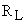for maximum power transfer.  What will be the value of maximum power?                                (8)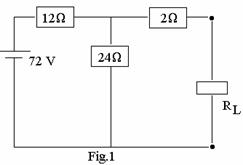Q.3     a.   A series circuit of resistance 250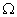and inductance 0.25 H is excited from a pulse voltage of strength 10 V of duration 1 ms. Find the value of the current at 0.5 ms and 2 ms.                                      (8)

b.   Find the average value of a full wave rectified sine wave shown in Fig.2.              (4)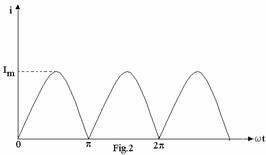c.   The electric mains in a house is marked as 230 V, 50 Hz.  Write down the equation for instantaneous voltage in sinusoidal form.                                (4)

Q.4     a.   How does the three – phase transformer differ from a single – phase one.  Give advantages and disadvantages of a 3 – phase transformer.                                                           (8)

b.   A 3 – phase transformer consisting of three 1 – phase transformers each with turn ratio of 10:1 (primary : secondary) is used to supply a 3 – phase load of 120 kVA at 400 V on the secondary side.  Calculate the primary line current and voltage if the transformer is connected   (i)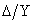(ii)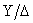.  What is the line-line transformation ratio in each case?         (8)

Q.5     a.   Briefly describe the different types of dc motors and give their connection diagrams.            (8)

b.   A separately excited dc motor is operating at an armature voltage of 300 V.  It’s no-load speed is 1200 rpm.  When fully loaded it delivers a motor torque of 350 N-m and its speed drops to 1100 rpm.  What is the full load current and power?  What is the armature resistance of the motor?  The motor is now fed with an armature voltage of 600 V, while its excitation is held fixed as before.  If it is once again fully loaded, find the motor torque, power and speed.                                                                  (8)

Q.6     a.   Explain, the construction, working principle & applications of a single-phase induction motor.                                                                     (8)

b.   A 3-phase induction motor which is wound for 4-poles, when running on full load, develops a useful torque of 100 Nm; also the rotor emf is observed to make 120-cycles/ min.  It is known that the torque lost on account of friction and core loss is 7 Nm.  Calculate the shaft power output, rotor copper loss, motor input and motor efficiency.                            (8)

Q.7     a.   Explain how motors are selected for specific engineering applications.                 (8)

b.   Explain the uses of:  shaded – pole motor, capacitor start motor, DC series motor and DC shunt motor.                                                                 (8)

Q.8     a.   Explain the following terms – Busbar, load, system, outage.                                 (8)

b.   A generating station has a maximum demand of 25 MW, a load factor of 60%, a plant capacity factor of 50% and a plant use factor of 72%.  Find

(i)  the daily energy produced               (ii)  reserve capacity of the plant,

(iii) the maximum energy that could be produced daily, if the plant, while running as per schedule, were fully loaded.                                                (8)

Q.9     a.   Explain the construction of a lead acid battery and give the equations during the charging and discharging process.                                              (8)

b.   Calculate the maximum power by a solar cell at an intensity of 200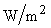.  Given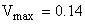V and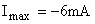.  Also calculate the cell efficiency if the area is 4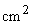.                               (8)